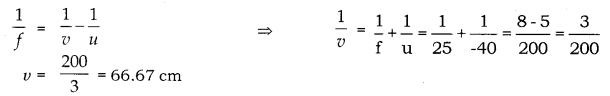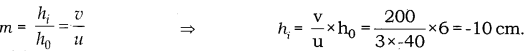# A 6 cm tall object is placed perpendicular to the principal axis of a convex lens of focal length 25 cm

A 6 cm tall object is placed perpendicular to the principal axis of a convex lens of focal length 25 cm. The distance of the object from the lens is 40 cm.
By calculation determine :
(a) the position and
(b) the size of the image formed.

Given, Height of an object h0 = 6 cm
Focal length of a lens, f = 25 cm
Distance of an object, u = - 40 cm
(a) Using lens formula,(b) Magnification